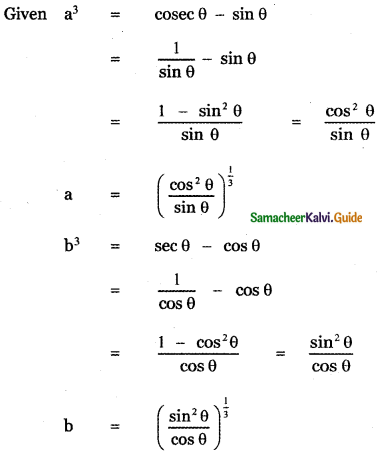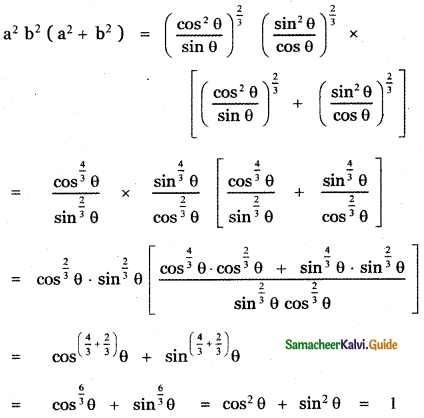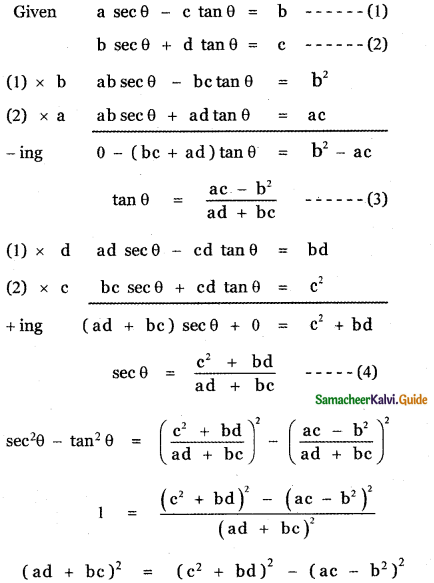Tamilnadu State Board New Syllabus Samacheer Kalvi 11th Maths Guide Pdf Chapter 3 Trigonometry Ex 3.1 Text Book Back Questions and Answers, Notes.

## Tamilnadu Samacheer Kalvi 11th Maths Solutions Chapter 3 Trigonometry Ex 3.1

Question 1.
Identify the quadrant in which an angle of each given measure lies,
(i) 25°
(ii) 825°
(iii) – 55°
(iv) 328°
(v) – 230°
(i) 25°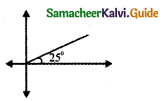(ii) 825°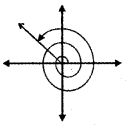825° = 9 × 90° + 15°
825° = 2 × 360° + 105°
∴ 825° lies in the second quadrant.

iii) -55°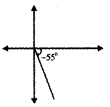-55° lies in the fourth quadrant

iv) 328°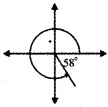328° = 270° + 58° lies in the fourth quadrant.

v) -230°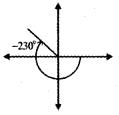– 230° = – 180° + (- 50°) lies in the second quadrant.Question 2.
For each given angle, find a co-terminal angle with a measure of 9 such that 0 ≤ θ < 360°.
(i) 395°
(ii) 525°
(iii) 1150°
(iv) – 270°
(v) – 450°
(i) 395°
395° = 360° + 35°
395° – 35° = 360°
∴ Coterminal angle for 395° is 35°

(ii) 525°
525° = 360° + 165°
360° – 165° = 360°
∴Coterminal angle for 525° is 165°

(iii) 1150°
1150° = 360° + 360° + 360° + 70°
1150° = 3 × 360° + 70°
1150° – 70° = 3 × 360°
∴ Coterminal angle for 1150° is 70°.

(iv) – 270°
– 270° = 360° + 90°
– 270° – 90° = 360°
∴ Coterminal angle for -270° is 90°

(v) – 450°
– 450° = – 720° + 270°
– 450° – 270° = – 2 × 360°
∴ Coterminal angle for – 450° is 270°Question 3.
If a cos θ – b sin θ = c , show that a sin θ + b cos θ = ± $$\sqrt{\mathbf{a}^{2}+\mathbf{b}^{2}-\mathbf{c}^{2}}$$
a cos θ – b sin θ = c
(a cos θ – b sin θ)2 + (a sin θ + b cos θ)2 = a2 cos2 θ – 2 ab sin θ cos θ + b2 sin2θ + a2 sin2 θ + b2 cos2 θ + 2 ab sin θ cos θ
c2 + (a sin 0 + b cos θ )2 = a2 cos2 θ + a2 sin2 θ + b2 sin2 θ + b2cos2θ
= a2 (cos2θ + sin2θ) + b2(sin2θ + cos2θ)
c2 + (a sin θ + b cos θ )2 = a2 + b2
(a sin θ + b cos θ)2 = a2 + b2 – c2
a sin θ + b cos θ = ± $$\sqrt{\mathbf{a}^{2}+\mathbf{b}^{2}-\mathbf{c}^{2}}$$

Question 4.
If sin θ + cos θ = m , show that cos6 θ + sin6 θ = $$\frac{4-3\left(m^{2}-1\right)^{2}}{4}$$ where m2 ≤ 2.
sin θ + cos θ = m
(sin θ + cos θ)2 = m2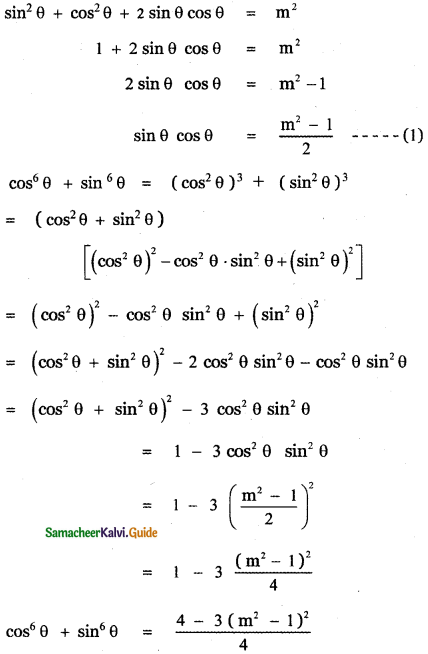Question 5.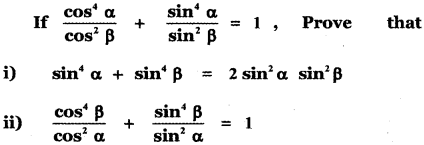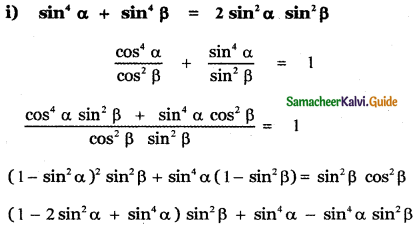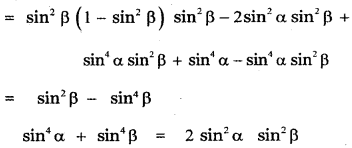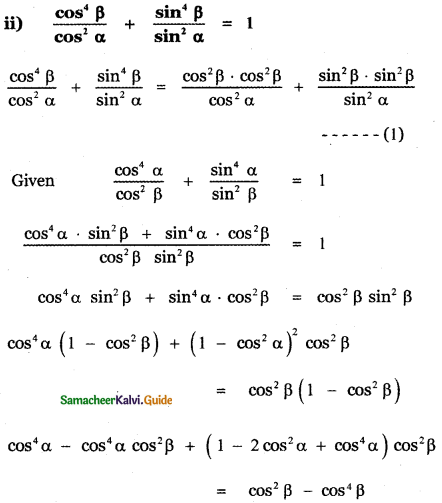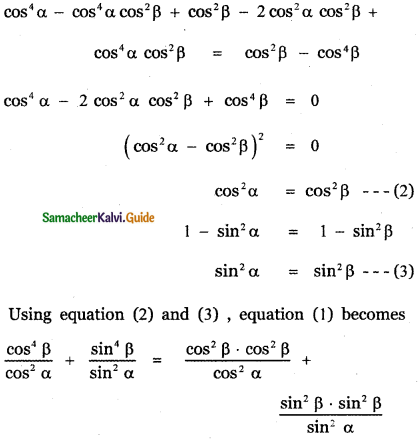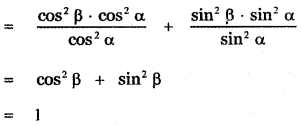Question 6.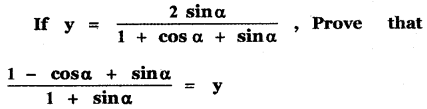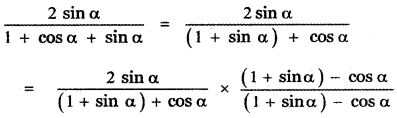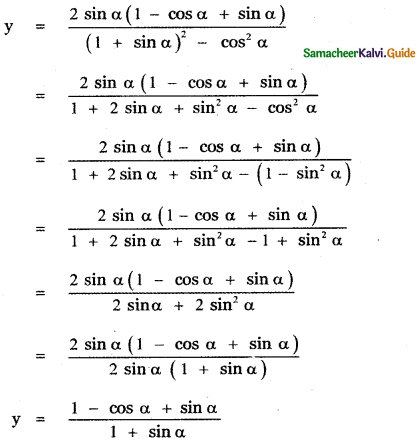Question 7.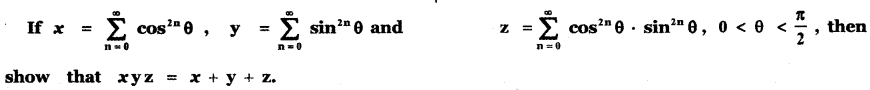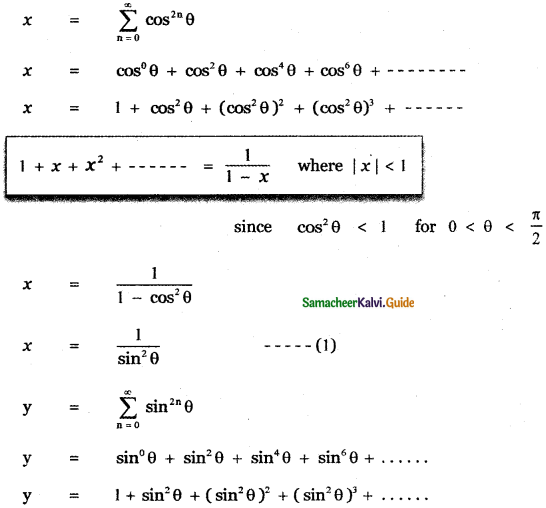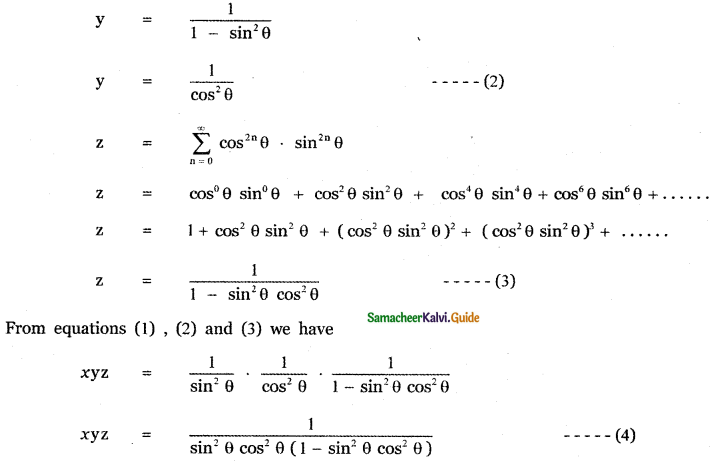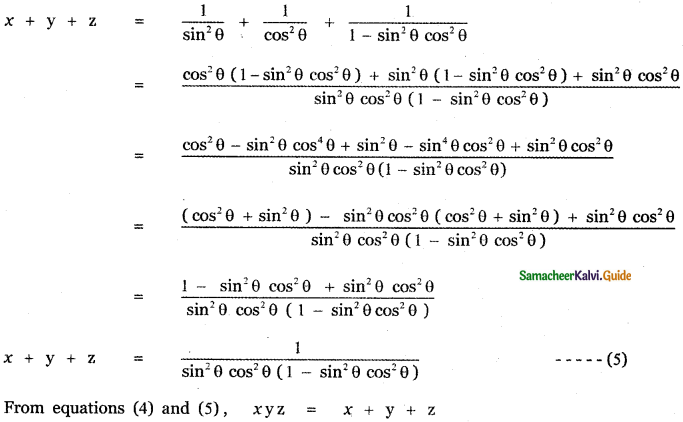Question 8.
If tan2 θ = 1 – k2, show that sec θ + tan3 θ cosec θ = ( 2 – k2)3/2. Also, find the values of k for which this result holds.
tan2 θ = 1 – k2
1 + tan2 θ = 1 + 1 – k2
sec2θ = (2 – k2)
sec2θ = (2 – k2)1/2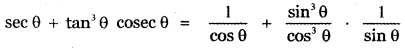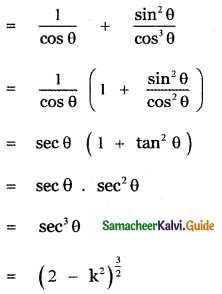tan2 θ = 1 – k2
When θ = $$\frac{\pi}{2}$$, tan $$\frac{\pi}{2}$$ = ∞, not defined 2
When θ = 0, tan2 0 = 1 – k2
1 – k2 = 0 ⇒ k2 = 1 ⇒ k = ± 1
When θ = 45°, tan2 45° = 1 – k2
1 – k2 = 1 ⇒ – k2 = 0 ⇒ k = 0
When θ > 45°, say θ = 60°
tan2 60° = 1 – k2 = (√3)2 = 1 – k2
3 = 1 – k2 ⇒ k2 = 1 – 3 = – 2
∴ θ > 45°, k2 is negative ⇒ k is imaginary
∴ k lies between -1 and 1 ⇒ k ∈ [-1 , 1]Question 9.
If sec θ + tan θ = p, obtain the values of sec θ, tan θ and sin θ in terms of p.
Given sec θ + tan θ = p
We have sec2 θ – tan2 θ = 1
(sec θ + tan θ) (sec θ – tan θ) = 1
p (sec θ – tan θ) = 1
sec θ – tan θ = $$\frac{1}{p}$$
(sec θ – tan θ) + (sec θ – tan θ) = p + $$\frac{1}{p}$$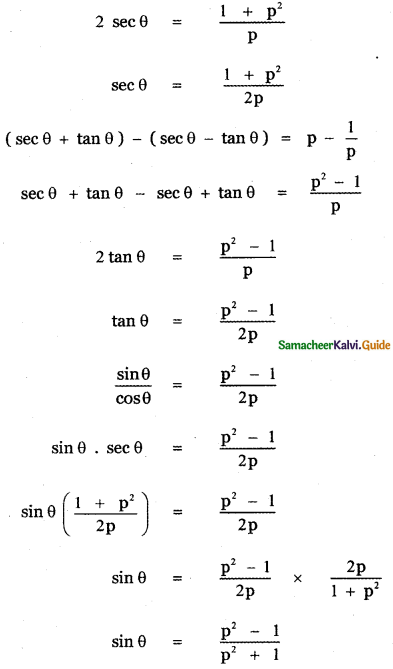Question 10.
If cot θ(1 + sin θ) = 4m and cot θ (1 – sin θ) = 4n then prove that (m2 – n2)2 = mn.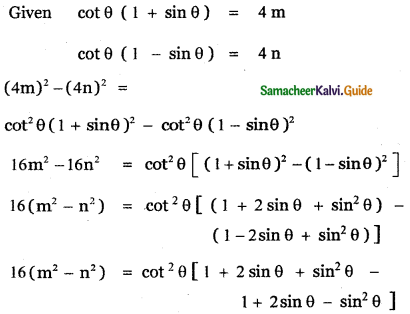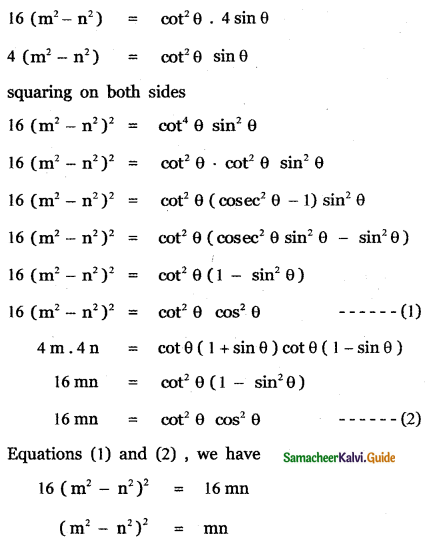Question 11.
If cosec θ – sin θ = a3, sec θ – cos θ = b3 then prove that a2b2(a2 + b2) = 1.